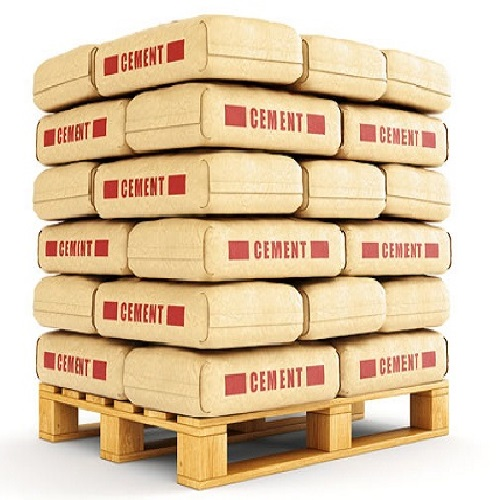#### Construction Cost Calculator :

Construction Cost
Builtup Area (Sq.ft) Approx Cost (Per Sq.ft) Approx amount of construction is :Cement Required :Cement Amount :Sand Required :Sand Amount :Aggregate Required :Aggregate Amount :Steel Required :Steel Amount :Paint Required :Bricks Required :Floorings : Finishers : Fittings :
##### Construction Cost :
• In this , Construction Cost Calculator we can used to calculate the Total Amount , Cement Required and Amount , Sand Required and Amount , Aggregate Required and Amount , Steel Required and Amount , Paint and Brick Required , Floorings , Finishers and Fittings.
##### Construction Cost Calculation Formula :
• To find the cost.
• Cost = Builtup area * Approx cost.
• Cement Required = Builtup area * 0.4.
• Cement Amount = (16.4/100) * Approx amount of cost.
• Sand Required = Builtup Area * 0.816.
• Sand Amount = (12.3/100) * Approx amount of cost.
• Aggregate Required = Builtup Area * 0.608.
• Aggregate Amount = (7.4/100) * Approx amount of cost.
• Steel Required = Builtup Area * 4.
• Steel Amount = (24.6/100) * Approx. amount of cost.
• Paint Required = Builtup Area * 0.18.
• Brick Required = Builtup Area * 8.
• Floorings = Builtup Area * 1.3.
• Finishers = (16.5 / 100) * Approx amount of cost.
• Fittings = (22.8 / 100) *Approx amount of cost.
##### How to Calculate ?
• Enter the Builtup Area of sq.ft.
• Enter the Approx Cost per sq.ft.
• Click “Calculate” button to get the result.
• Click “Reset” to clear all the values.
Hi Myself Raj,
Do you need any BIM Modelling Services